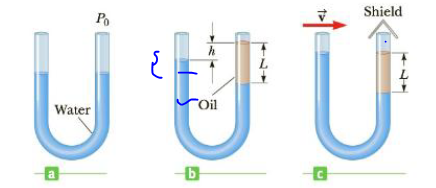Chapter 9, Problem 88AP

Chapter
Section
Textbook Problem

A U-tube open at both ends is partially filled with water (Fig. P9.88a). Oil (ρ = 750 kg/m3) is then poured into the right arm and forms a column L = 5.00 cm high (Fig. P9.88b). (a) Determine the difference h in the heights of the two liquid surfaces, (b) The right arm is then shielded from any air motion while air is blown across the top of the left arm until the surfaces of the two liquids are at the same height (Fig. P9.88c). Determine the speed of the air being blown across the left arm. Assume the density of air is 1.29 kg/m3.Figure P9.88

(a)

To determine
The difference h in the height of the two liquid surfaces.

Explanation
The pressures at both points A and B as shown in figure are equal. The pressure at point A is PA=Patm+ρwaterg(Lh) and the pressure at point B is PB=Patm+ρoilgL but we know that pressures at PA=PB . By solving these two expressions, the difference in has been calculated.

Given info: Density of oil is 750kg/m3 , density of water is 1.00×103kg/m3 , and the height of the oil in the column is 5.00cm .

The formula for the difference h in the height of the two liquid surfaces is,

h=(1ρoilρwater)L

• ρoil is density of oil.
• ρwater is density of water

(b)

To determine
The speed of the air being blown across the left arm.

Still sussing out bartleby?

Check out a sample textbook solution.

See a sample solution

The Solution to Your Study Problems

Bartleby provides explanations to thousands of textbook problems written by our experts, many with advanced degrees!

Get Started

What is the name of the element in Group 4A and Period 5?

General Chemistry - Standalone book (MindTap Course List)

The energy intake recommendation is set at a level predicted to maintain body weight. T F

Nutrition: Concepts and Controversies - Standalone book (MindTap Course List)

Suggest a way to cure kwashiorkor.

Introduction to General, Organic and Biochemistry